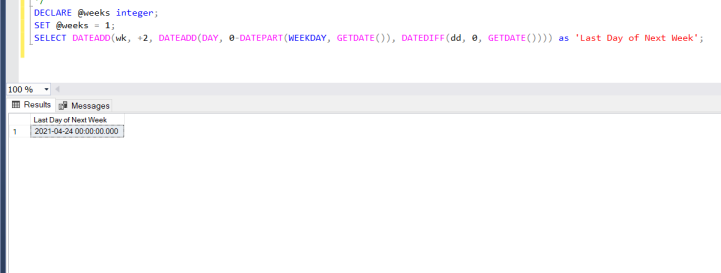# SQL Query to Get First and Last Day of a Week in a Database

An SQL query is a single line statement of a Program written in a particular language to perform a specific task. A query consists of some pre-defined functions in SQL like SELECT, CREATE, etc. So, in this article, we will learn about SQL query to get the first and last day of a week in a database. So let’s create a database first to do our task, here we will be using Microsoft SQL Server:

Step 1: Create a database named as GFG:

`CREATE DATABASE GFG`

Step 2: Use this database:

`USE GFG`

Step 3: Create a table in this database:

```CREATE TABLE geeksforgeeks(
id integer,
FirstDay varchar(20)
)```

Describe this table:

`sp_help 'dbo.geeksforgeeks'`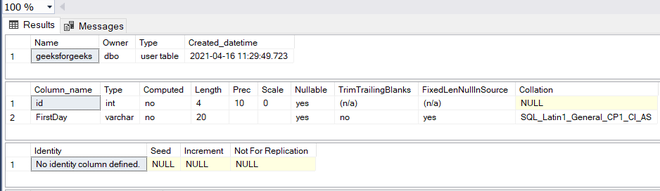Step 4: Insert the values of dates in this database:

```INSERT INTO [dbo].[geeksforgeeks]
([id] ,[FirstDay])
VALUES
(1, 'Monday')
GO

INSERT INTO [dbo].[geeksforgeeks]
([id] ,[FirstDay])
VALUES
(2, 'Tuesday')
GO

INSERT INTO [dbo].[geeksforgeeks]
([id] ,[FirstDay])
VALUES
(3, 'Wednesday')
GO

INSERT INTO [dbo].[geeksforgeeks]
([id] ,[FirstDay])
VALUES
(4, 'Thursday')
GO

INSERT INTO [dbo].[geeksforgeeks]
([id] ,[FirstDay])
VALUES
(5, 'Friday')
GO

INSERT INTO [dbo].[geeksforgeeks]
([id] ,[FirstDay])
VALUES
(6, 'Saturday')
GO

INSERT INTO [dbo].[geeksforgeeks]
([id] ,[FirstDay])
VALUES
(7, 'Sunday')
GO```

Now, we are checking that the data is inserted successfully or not:

`SELECT * FROM [dbo].[geeksforgeeks]`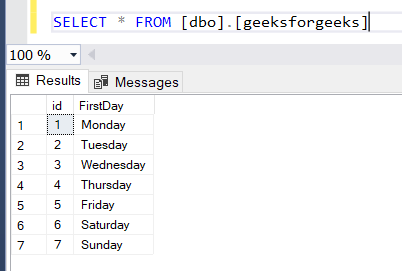Step 5: Now check the first day of the week:

`SELECT id, FirstDay FROM [dbo].[geeksforgeeks] WHERE id = @@DATEFIRST `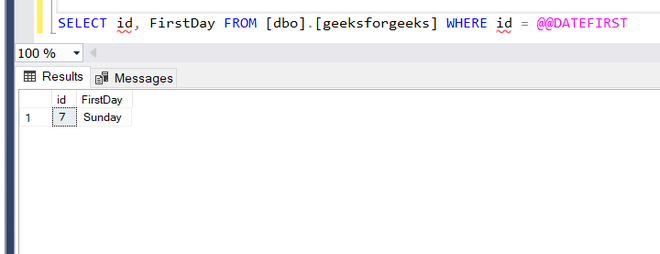So we can see the first day of the week is Sunday

Step 6: Now we will find out what is the first day of the next week using the DATEADD function():

```/*
If you want to find out the first day of
upcoming weeks then set @weeks as a positive integer
with number of weeks, else negative integer.
*/

DECLARE @weeks integer;
SET @weeks = 1;
DATEDIFF(dd, 0, GETDATE()))) as 'First Day of next week';```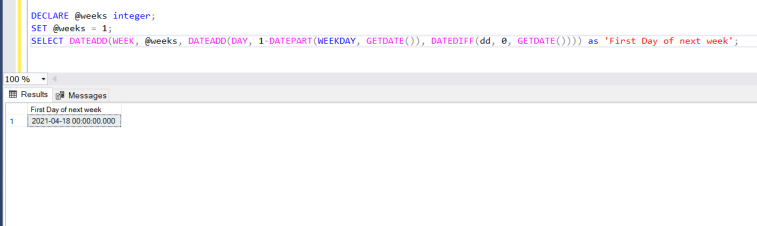Step 7: Now we will find out what is the last day of the next week using the DATEADD function:

```/*
If you want to find out the first day of
previous weeks then set @weeks as a negative integer
with number of weeks, else negative integer.
*/

DECLARE @weeks integer;
SET @weeks = 1;